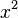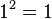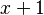# Fixed point facts for kids

Kids Encyclopedia Facts

A value x is a fixed point of a function f if and only if f(x) = x.

## Examples

1 is a fixed point of$x^2$ because$1^2 = 1$. Some functions do not have fixed points. For example$x + 1$ does not have one because$x + 1$ is never equal to x.Fixed point Facts for Kids. Kiddle Encyclopedia.